# 零基础入门深度学习(3) - 神经网络和反向传播算法

## 神经元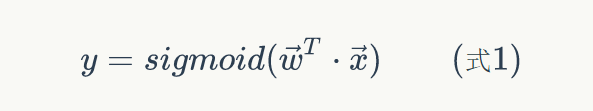sigmoid函数的定义如下：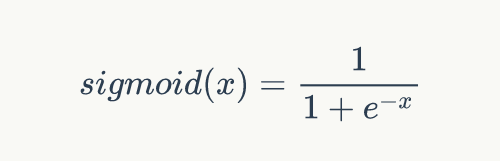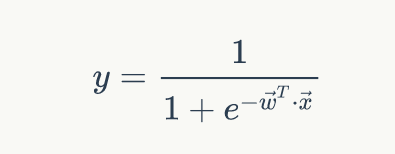sigmoid函数是一个非线性函数，值域是(0,1)。函数图像如下图所示sigmoid函数的导数是：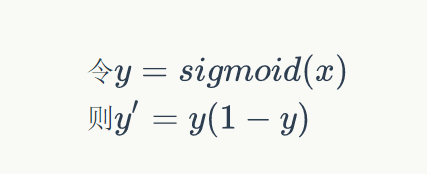## 神经网络是啥• 神经元按照来布局。最左边的层叫做输入层，负责接收输入数据；最右边的层叫输出层，我们可以从这层获取神经网络输出数据。输入层和输出层之间的层叫做隐藏层，因为它们对于外部来说是不可见的。
• 同一层的神经元之间没有连接。
• 第N层的每个神经元和第N-1层的所有神经元相连(这就是full connected的含义)，第N-1层神经元的输出就是第N层神经元的输入。
• 每个连接都有一个权值

## 计算神经网络的输出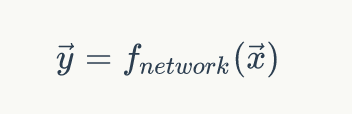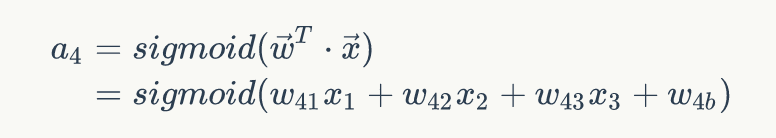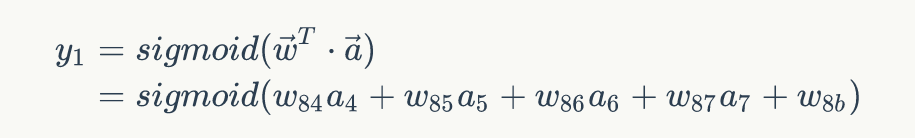### 神经网络的矩阵表示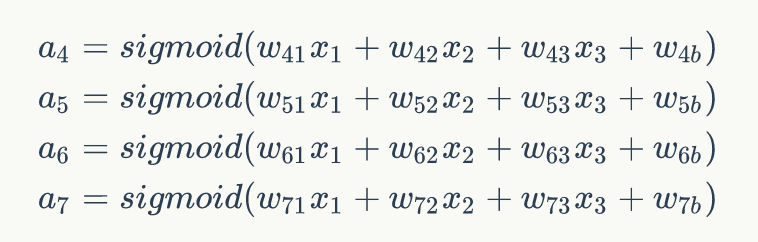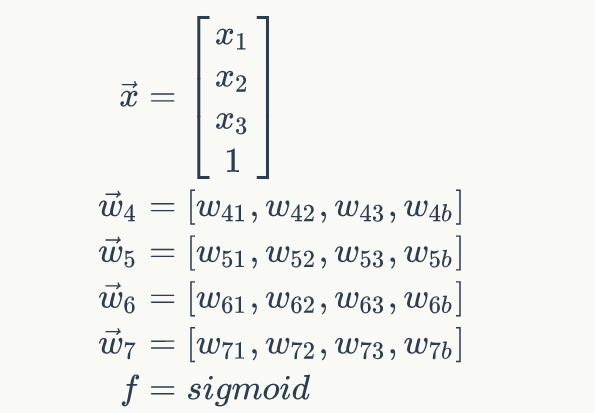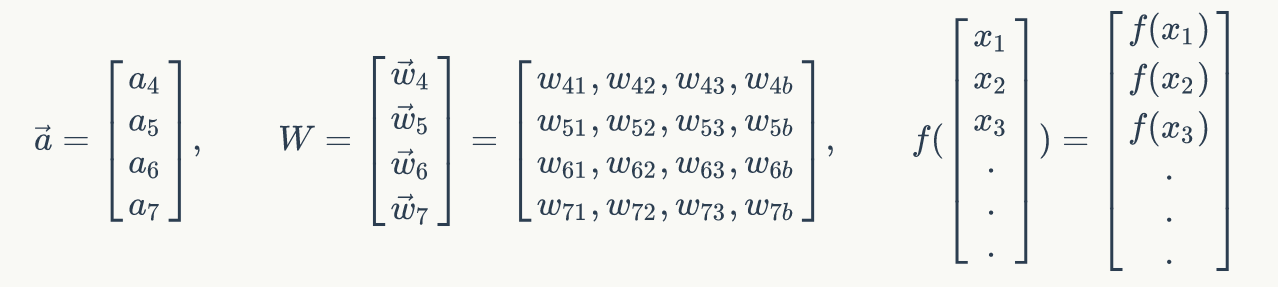## 神经网络的训练

### 反向传播算法(Back Propagation)• 对于输出层节点i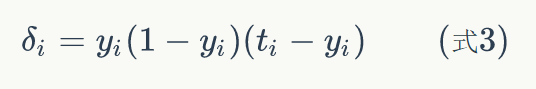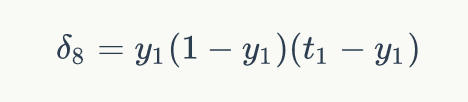• 对于隐藏层节点，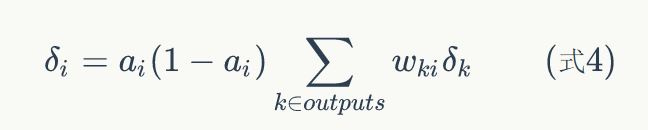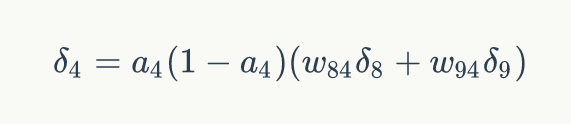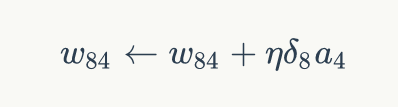### 超参数的确定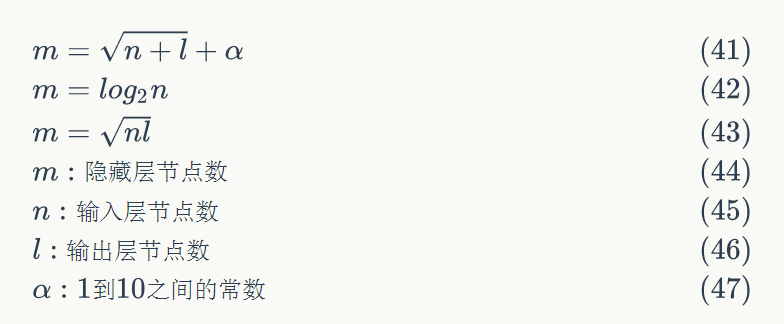## 向量化编程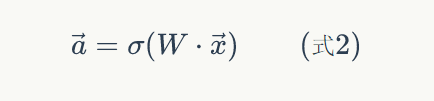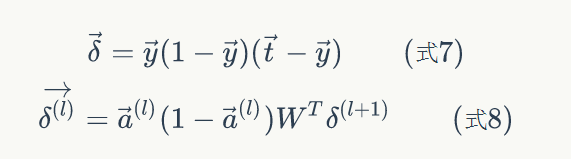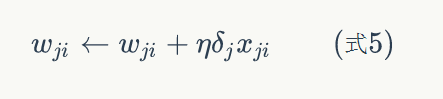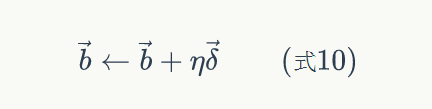# 2. 当为mat的时候，默认d*f就是矩阵的乘积，multiply转化为对应元素的乘积，dot（d,f）为矩阵的乘积。

# 全连接每层的实现类。输入对象x、神经层输出a、输出y均为列向量
class FullConnectedLayer(object):
# 构造函数。input_size: 本层输入向量的维度。output_size: 本层输出向量的维度。activator: 激活函数
def __init__(self, input_size, output_size,activator):
self.input_size = input_size
self.output_size = output_size
self.activator = activator
# 权重数组W
self.W = np.random.uniform(-0.1, 0.1,(output_size, input_size))  #初始化为-0.1~0.1之间的数。权重的大小。行数=输出个数，列数=输入个数。a=w*x，a和x都是列向量
# 偏置项b
self.b = np.zeros((output_size, 1))  # 全0列向量偏重项
# 输出向量
self.output = np.zeros((output_size, 1)) #初始化为全0列向量

# 前向计算，预测输出。input_array: 输入向量，维度必须等于input_size
def forward(self, input_array):   # 式2
self.input = input_array
self.output = self.activator.forward(np.dot(self.W, input_array) + self.b)

# 反向计算W和b的梯度。delta_array: 从上一层传递过来的误差项。列向量
def backward(self, delta_array):
# 式8
self.delta = np.multiply(self.activator.backward(self.input),np.dot(self.W.T, delta_array))   #计算当前层的误差，已被上一层使用
self.W_grad = np.dot(delta_array, self.input.T)   # 计算w的梯度。梯度=误差.*输入
self.b_grad = delta_array  #计算b的梯度

# 使用梯度下降算法更新权重
def update(self, learning_rate):
self.W += learning_rate * self.W_grad
self.b += learning_rate * self.b_grad

# Sigmoid激活函数类
class SigmoidActivator(object):
def forward(self, weighted_input): #前向传播计算输出
return 1.0 / (1.0 + np.exp(-weighted_input))
def backward(self, output):  #后向传播计算w和b的梯度
return np.multiply(output,(1 - output))   # 对应元素相乘

# 神经网络类
class Network(object):
# 初始化一个全连接神经网络。layers:数组，描述神经网络每层节点数。包含输入层节点个数、隐藏层节点个数、输出层节点个数
def __init__(self, layers):
self.layers = []
for i in range(len(layers) - 1):
self.layers.append(FullConnectedLayer(layers[i], layers[i+1],SigmoidActivator()))   # 创建全连接层，并添加到layers中

# 训练函数。labels: 样本标签矩阵。data_set: 输入样本矩阵。rate: 学习速率。epoch: 训练轮数
def train(self, labels, data_set, rate, epoch):
for i in range(epoch):
for d in range(len(data_set)):
self.train_one_sample(labels[d].reshape(-1,1),data_set[d].reshape(-1,1), rate)   #将输入对象和输出标签转化为列向量

# 内部函数，用一个样本训练网络
def train_one_sample(self, label, sample, rate):
# print('样本：\n',sample)
self.predict(sample)  # 根据样本对象预测值
self.calc_gradient(label) # 计算梯度
self.update_weight(rate) # 更新权重

# 使用神经网络实现预测。sample: 输入样本
def predict(self, sample):
sample = sample.reshape(-1,1)   #将样本转换为列向量
output = sample  # 输入样本作为输入层的输出
for layer in self.layers:
# print('权值：',layer.W,layer.b)
layer.forward(output)  # 逐层向后计算预测值。因为每层都是线性回归
output = layer.output
# print('预测输出：', output)
return output

# 内部函数，计算每个节点的误差。label为一个样本的输出向量，也就对应了最后一个所有输出节点输出的值
def calc_gradient(self, label):
# print('计算梯度：',self.layers[-1].activator.backward(self.layers[-1].output).shape)
delta = np.multiply(self.layers[-1].activator.backward(self.layers[-1].output),(label - self.layers[-1].output))  #计算输出误差
# print('输出误差：', delta.shape)
for layer in self.layers[::-1]:
layer.backward(delta)   # 逐层向前计算误差。计算神经网络层和输入层误差
delta = layer.delta
# print('当前层误差：', delta.shape)
return delta

# 内部函数，更新每个连接权重
def update_weight(self, rate):
for layer in self.layers:  # 逐层更新权重
layer.update(rate)


#由于使用了逻辑回归函数，所以只能进行分类识别。识别ont-hot编码的结果
if __name__ == '__main__':
# 使用神经网络实现and运算
data_set = np.array([[0,0],[0,1],[1,0],[1,1]])
labels = np.array([[1,0],[1,0],[1,0],[0,1]])
# print(data_set)
# print(labels)
net = Network([2,1,2])  # 输入节点2个（偏量b会自动加上），神经元1个，输出节点2个。
net.train(labels, data_set, 2, 100)
for layer in net.layers:  # 网络层总不包含输出层
print('W:',layer.W)
print('b:',layer.b)

# 对结果进行预测
sample = np.mat([[0,1]])
y = net.predict(sample)
print(y)